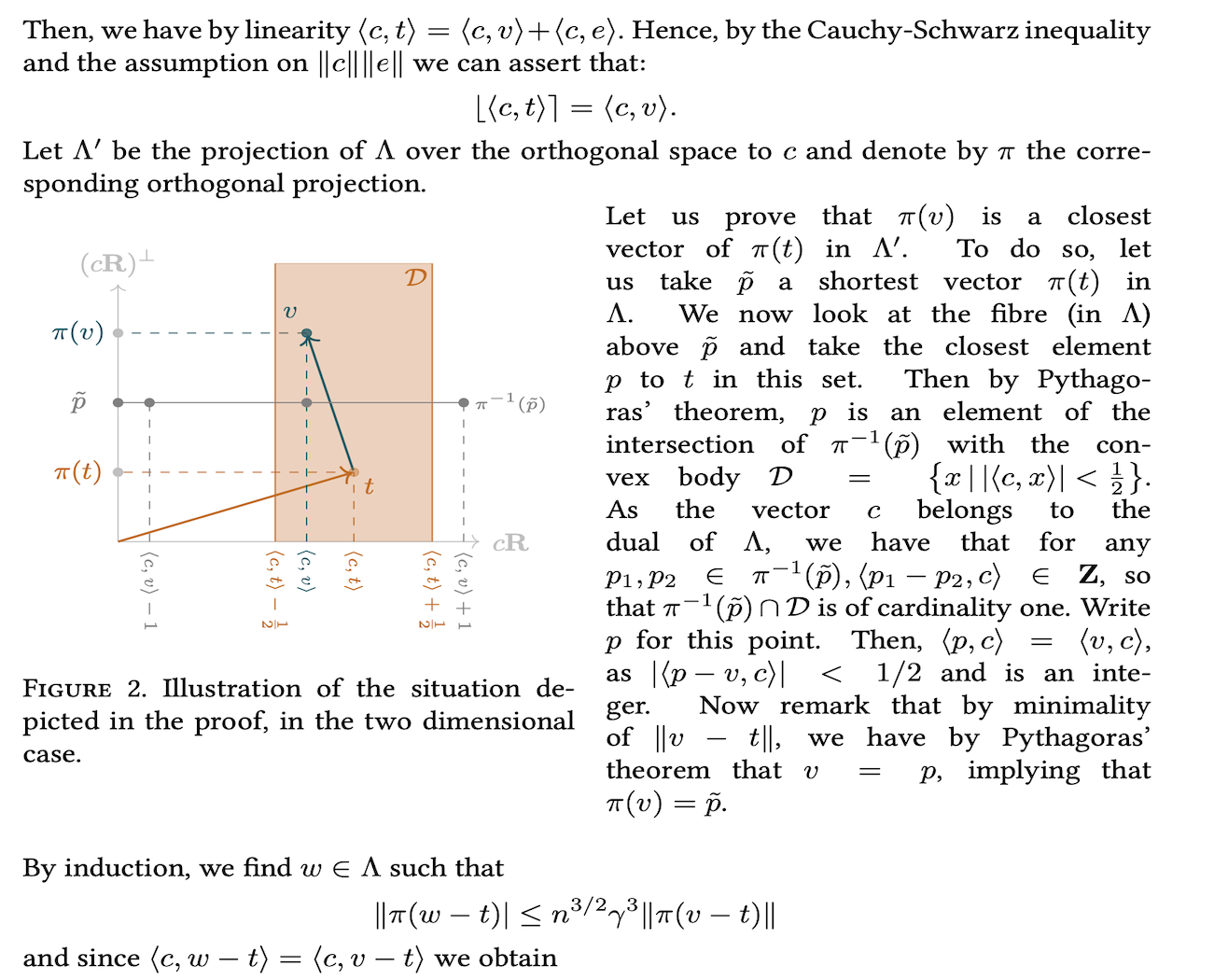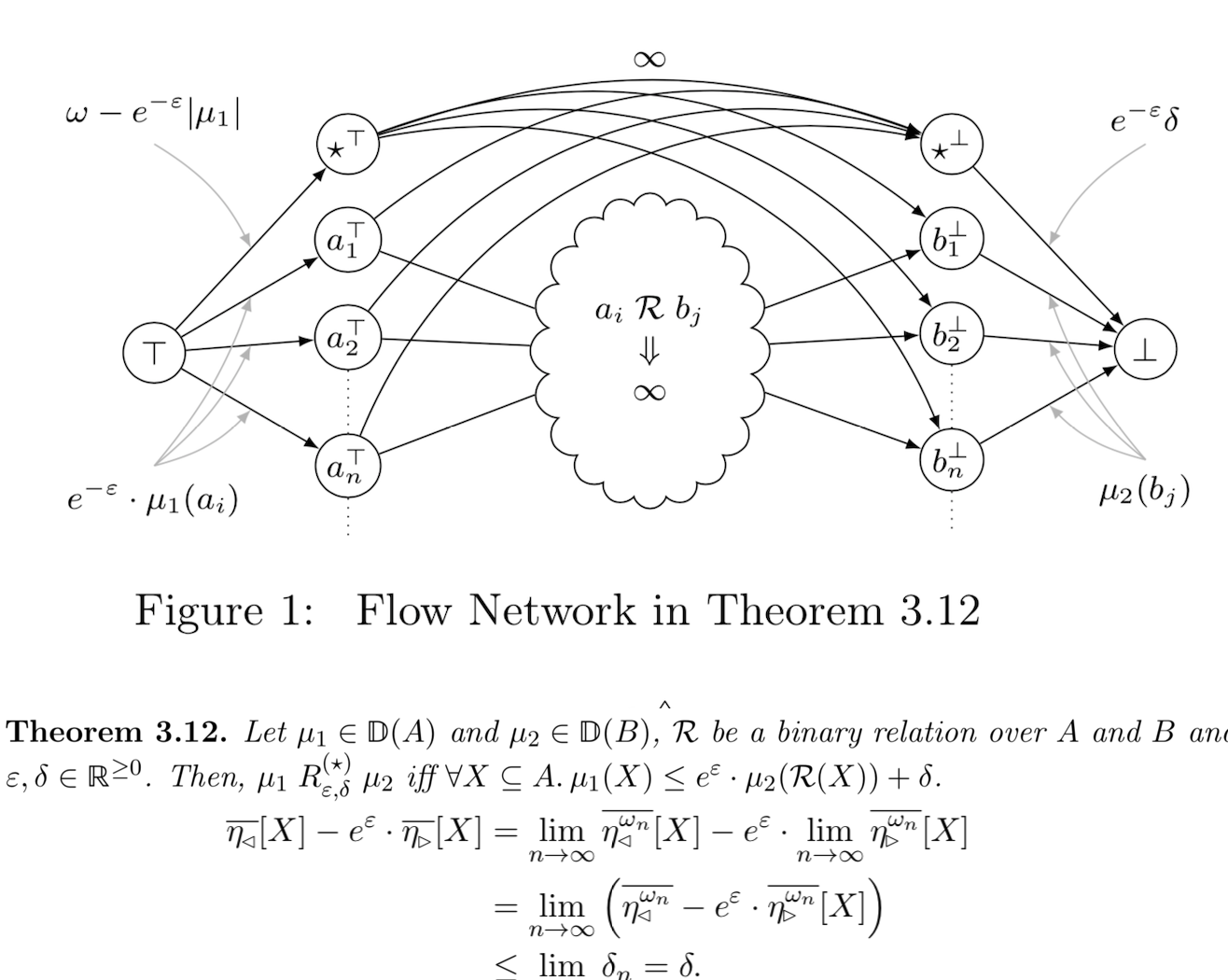I'm Thomas Espitau - エスピト - currently Postdoc at NTT Secure Platform Laboratories, in Tokyo. I pursued my Ph.D. in algorithmic number theory at Sorbonne University in Paris, advised by prof. Antoine Joux and prof. Pierre-Alain Fouque. I love maths, computer science, skiing and surfing. I'm also an enthusiastic Kenshi and Go player.

### Research Interests

##### Geometry of numbers and lattices
• Theta function of lattices
• Convex geometry
• Discrete geometry
##### (Algorithmic) Number theory
• Reduction of vector bundles over arithmetic curves curves
• Class group computation
• Effective Arakelov theory
##### Cryptography
• Lattice-based cryptography
• Statistical learning and cryptanalysis
• Secure and efficient implementation
##### Program verification
• Verification of probabilistic programs
• Coupling-based techniques
• Relational methods

### Most Recent Work           All Publications### The nearest-colattice algorithm: time-approximation tradeoff for approx-CVP

We exhibit a hierarchy of polynomial time algorithms solving approximate variants of the Closest Vector Problem. Our contributions is on the one hand a heuristic algorithm achieving the same distance as HSVP algorithms, and on the other hand a proven reduction from approximating the closest vector with a factor $$\approx n^{\frac32}\beta^{\frac{3n}{2\beta}}$$ to the Shortest Vector Problem in dimension $$\beta$$.### Relational -Liftings for Differential Privacy

We propose a novel, existential version of approximate lifting, called ⋆-lifting, and show that it is equivalent to Sato's construction for discrete probability measures.We also clarify the relation between existing definitions of approximate lifting, and consider more general approximate liftings based on f-divergences.